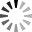# 17. Indices and multiple security strategies - 1.

17. Indices and multiple security strategies - 1.VideoUnavailable
The lesson covers:
• Considered working with stock indices
• Considered working with futures on the example of a strategy with conditions:
If the index calculated by the SBER @ TQBR / SRM9 @ FORTS formula is less than the average value for 10 periods, then Sberbank shares are sold.
If the index calculated by the SBER @ TQBR / SRM9 @ FORTS formula is greater than the average value for 10 periods, then we buy Sberbank shares.

• The function of constructing a continuous futures is considered
• A strategy with a continuous futures is built with the condition:
If the current value of the SBER @ TQBR / SBER_СF @ FORTS index is 0.005 units above the average, sell the instrument Sberbank Shares and buy the instrument of Sberbank shares.
If the current value of the SBER @ TQBR / SBER_СF @ FORTS index is 0.005 units lower than the average, buy the Sberbank Shares instrument and sell the Sberbank shares instrument.

• Based on the strategy, an example of building a scheme with cancellation of an order is considered

 Спасибо:

Добавить файлы через драг-н-дроп, , или вставить из буфера обмена.